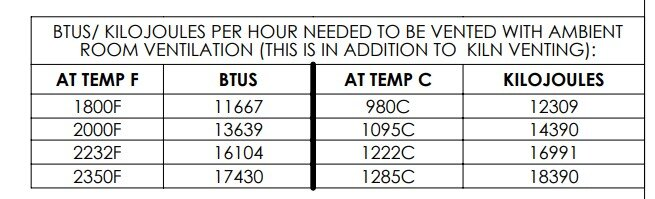# KNOWLEDGEBASE

### Calculating ventilation requirements for heat from a kiln room

#### Calculate how many CFM of air ventilation you need

1. Look up the number of BTUs/Hour that your kiln gives off. See the BTU chart located on the General Dimension Drawing of the kiln model. You can download the General Dimension Drawing from the web page for that kiln model. (See item #3 below for Easy-Load kilns).2. You will find a chart like this on the drawing:3. For Easy-Load kilns use the chart found in this PDF.
4. Use the BTU/Hour figure based on the maximum temperature you will be firing at. You can extrapolate these numbers. For instance, you can pick a BTU/Hour number between the column for 2232°F and 2350°F for Cone 8 firing.
5. The maximum temperature in the room is 120°F. This is below the safe limitation of the control and is probably as hot as you will want it to be in the room anyway.
6. Figure out what your typical hottest day is. You may decide not to fire your kiln, for instance, when it is 105°F outside and you will use something like 100°F as this design temperature.
7. This gives you a Delta T (temperature differential) of 20°F in this example.
8. Divide the BTU/Hour that you want to remove from the room by the product of 1.085 x the Delta T.

#### The Formula

(BTU per Hour) / (1.085 X DELTA T) = CFM

#### An Example

Calculate the ventilation CFM required for an e23T-3 kiln firing at Cone 6 with an outside temperature of 100°F.

1. Look up the BTU/Hour heat loss for an e23T-3 firing at Cone 6. According to the chart on the General Dimension Drawing the e23T-3 will give off 16,104 BTUs per hour at 2232F (Cone 6).
2. From above we have a Delta T of 20°F (120°F - 100°F)
3. 16,104/ (1.085 X 20) = 742 CFM
4. Size your ventilation fan for something above that number.
5. Make sure you have the ability to have makeup air get into the room (i.e. an air inlet into the room, open door or window). This will keep the static pressure to a minimum. An increase in static pressure will downrate the rated CFM of a given fan.